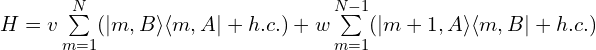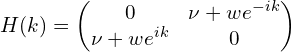# SSH模型的哈密顿量、能带图和卷绕数（附Python代码）

### 1. 模型

SSH模型示意图如下：

SSH（Su-Schrieffer-Heeger）模型的哈密顿量为：### 2. 能带图

SSH模型哈密顿量经过傅里叶变换后，得到："""
This code is supported by the website: https://www.guanjihuan.com
The newest version of this code is on the web page: https://www.guanjihuan.com/archives/5025
"""

import numpy as np
import matplotlib.pyplot as plt
from math import *
import cmath

def hamiltonian(k):  # SSH模型
v=0.6
w=1
matrix = np.zeros((2, 2), dtype=complex)
matrix[0,1] = v+w*cmath.exp(-1j*k)
matrix[1,0] = v+w*cmath.exp(1j*k)
return matrix

def main():
k = np.linspace(-pi, pi, 100)
plot_bands_one_dimension(k, hamiltonian)

def plot_bands_one_dimension(k, hamiltonian):
dim = hamiltonian(0).shape
dim_k = k.shape
eigenvalue_k = np.zeros((dim_k, dim))
i0 = 0
for k0 in k:
matrix0 = hamiltonian(k0)
eigenvalue, eigenvector = np.linalg.eig(matrix0)
eigenvalue_k[i0, :] = np.sort(np.real(eigenvalue[:]))
i0 += 1
for dim0 in range(dim):
plt.plot(k, eigenvalue_k[:, dim0], '-k')
plt.show()

if __name__ == '__main__':
main()


### 3. 卷绕数

"""
This code is supported by the website: https://www.guanjihuan.com
The newest version of this code is on the web page: https://www.guanjihuan.com/archives/5025
"""

import numpy as np
import matplotlib.pyplot as plt
from math import *
import cmath
import time

def hamiltonian(k):  # SSH模型
v=0.6
w=1
matrix = np.zeros((2, 2), dtype=complex)
matrix[0,1] = v+w*cmath.exp(-1j*k)
matrix[1,0] = v+w*cmath.exp(1j*k)
return matrix

def main():
start_clock = time.perf_counter()
delta_1 = 1e-9  # 求导的步长（求导的步长可以尽可能短）
delta_2 = 1e-5  # 积分的步长（积分步长和计算时间相关，因此取一个合理值即可）
W = 0  # Winding number初始化
for k in np.arange(-pi, pi, delta_2):
H = hamiltonian(k)
log0 = cmath.log(H[0, 1])

H_delta = hamiltonian(k+delta_1)
log1 = cmath.log(H_delta[0, 1])

W = W + (log1-log0)/delta_1*delta_2 # Winding number
print('Winding number = ', W/2/pi/1j)
end_clock = time.perf_counter()
print('CPU执行时间(min)=', (end_clock-start_clock)/60)

if __name__ == '__main__':
main()


12,438 次浏览

【说明：本站主要是个人的一些笔记和代码分享，内容可能会不定期修改。为了使全网显示的始终是最新版本，这里的文章未经同意请勿转载。引用请注明出处：https://www.guanjihuan.com

## 22 thoughts on “SSH模型的哈密顿量、能带图和卷绕数（附Python代码）”

1.lhaaw说道：

老师您好，我试了一下，最后的卷绕数可以通过留数法解析求得，设z=e^(ik)，复平面存在两个奇点z=0和z=-w/v，卷绕数等于半径为1的C内的留数和，z=0和z=-w/v对应的留数分别为-1和1，若w>v，则留数和为-1；若w<v，则留数和为-1+1=0。

1.guanjihuan说道：

👍

2.jiang说道：

您好，在此模型中卷绕数是否与体系能量有关？卷绕数等于0是否代表体系能量平均分布，等于-1是否代表能量会聚集在某处？通过这个模型的哈密顿量来看，w, v的地位感觉上应该是等同的，为什么v>w时卷绕数会是0，w>v时卷绕数会是-1？而不是 1 和 -1？

1.guanjihuan说道：

（1）卷绕数跟体系能量无关。
（2）卷绕数是一种拓扑不变量，在有限长度时体现的是量子化的边缘态。跟能量平均分布没关系。
（3）如果是无限长的一维链，w和v的地位是相同的，所以在w和v对调后能带完全一样。在算卷绕数的时候，因为涉及到了元胞的选取，这已经包含了有限长度时边缘态的信息。卷绕数取的值跟定义有关，在不具有拓扑时，是定义为0。

2.guanjihuan说道：

极化跟wannier center的位置有关系，从实空间的这个角度去理解也可以。

1.jiang说道：

感谢大佬😊

3.cj说道：

请问数学上陈数应该是个整数才对，可是为什么代码算出来的陈数不是个整数捏（大二学生感到很迷惑）

1.guanjihuan说道：

在计算机中数字都是有位数的，例如单精度浮点数（float）最多有7位十进制有效数字，双精度浮点数（double）表示十进制的15或16位有效数字。在数值计算中，可能会遇到无理数，也会遇到除法运算的无限循环小数，都只是取了有限位数，多次计算后会积累不少误差，所以1e-6以下的值一般不会太准确。还有数值计算中的积分也只是用求和代替，并不是严格的积分。

4.匿名说道：

您好，方便问下哈密顿量是开放条件，那个能带图经过傅里叶变化的哈密顿量是在周期性边界条件下吗？(w项从m＝1到N)

1.guanjihuan说道：

能带图必须是在无限长的体系下，才会存在k。因为不是有限长的，所以没有开放条件，也没有周期性边界条件。
能带图k=0的情况，相当于元胞在周期性边界条件下的结果。

5.wang说道：

您好，这个程序只适用于手对称两能带系统是吗？

1.guanjihuan说道：

从定义来看，是只适用于手对称的系统，但不一定是两能带系统。
如果不满足手对称，可能有其他的拓扑不变量。

1.wang说道：

好的，谢谢

6.David说道：

请问高维度缠绕数的计算是需要先用投影算符降维吗？

1.guanjihuan说道：

没算过，不清楚。可以找下相关文献。

7.匿名说道：

你好，算winding number 的时候选取 的是h(k)，如果选取h(k)*的话计算结果是前者的相反数，请问应该选取哪一个？还是只关注其绝对值大小，选取任何一个都可以？

1.guanjihuan说道：

符号其实也跟积分的路径方向有关，一般会用来区分两个路径方向。其他时候只关注绝对值大小，负号影响不大。

8.匿名说道：

你好，我在求解winding number时，加入了一个循环，看u对于winding number的影响，大多数据很正常，为什么中间会出现一个两千多的数字呢？

1.guanjihuan说道：

是v=w的位置吗，这时候带隙为零，能带相交的地方波函数不好区分开。

9.yuan说道：

你好，我想问一下SSH模型那个哈密顿量您知道怎么推导出来的吗？一直很困惑

1.yuan说道：

我谷歌找到了understanding basic concepts of topological insulators through SSH model这篇论文，还找了其中的参考文献oxford solid state physics书，差不多弄懂了这个哈密顿量的来源。不用麻烦您了，打扰了。

1.guanjihuan说道：

嗯嗯，最明显的特征就是交替的跃迁项。